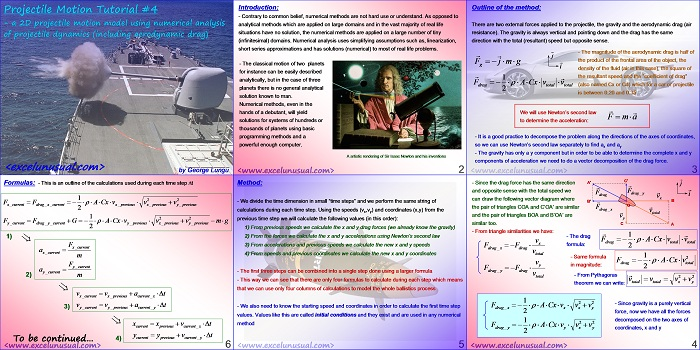# 2D Projectile Motion Tutorial #4

This tutorial derives the formulas of a projectile model taking into account the aerodynamic drag.

A finite differences numerical method is used. Though fairly easy to apply and understand, this type of methods can solve much more complex problems than the high-school type approach shown in the previous tutorials.

An Excel model will be implemented in the next section.by George Lungu

### Introduction:

– Contrary to common belief, numerical methods are not hard use or understand. As opposed to
analytical methods which are applied on large domains and in the vast majority of real life
situations have no solution, the numerical methods are applied on a large number of tiny
(infinitesimal) domains. Numerical analysis uses simplifying assumptions such as, linearization,
short series approximations and has solutions (numerical) to most of real life problems.

– The classical motion of two planets
for instance can be easily described
analytically, but in the case of three
planets there is no general analytical
solution known to man.

Numerical methods, even in the
hands of a debutante, will yield
solutions for systems of hundreds or
thousands of planets using basic
programming methods and a
powerful enough computer.

An artistic rendering of Sir Isaac Newton and his inventions

<www.excelunusual.com> 2

### Outline of the method:

There are two external forces applied to the projectile, the gravity and the aerodynamic drag (air
resistance). The gravity is always vertical and pointing down and the drag has the same
direction with the total (resultant) speed but opposite sense.

– The magnitude of the aerodynamic drag is half of the product of the frontal area of the object, the density of the fluid (air in this case), the square of
the resultant speed and the “coefficient of drag” (also named Cx or Cd) which for a car or projectile is between 0.20 and 0.35.

We will use Newton’s second law F = m*a to determine the acceleration:

– It is a good practice to decompose the problem along the directions of the axes of coordinates,
so we can use Newton’s second law separately to find ax and ay

– The gravity has only a y component but in order to be able to determine the complete x and y
components of acceleration we need to do a vector decomposition of the drag force.

– Since the drag force has the same direction and opposite sense with the total speed we can draw the following vector diagram where the pair of triangles
COA and C’OA’ are similar and the pair of triangles BOA and B’OA’ are similar too.

– From triangle similarities we have:

– The drag formula:

– Same formula 1
y in magnitude:
drag_ y drag
– From Pythagoras theorem we can write:

– Since gravity is a purely vertical force, now we have all the forces decomposed on the two axes of coordinates, x and y

<www.excelunusual.com> 4

### Method:

– We divide the time dimension in small “time steps” and we perform the same string of
calculations during each time step. Using the speeds (vx,vy) and coordinates (x,y) from the
previous time step we will calculate the following values (in this order):

1) From previous speeds we calculate the x and y drag forces (we already know the gravity)

2) From the forces we calculate the x and y accelerations using Newton’s second law

3) From accelerations and previous speeds we calculate the new x and y speeds

4) From speeds and previous coordinates we calculate the new x and y coordinates

– The first three steps can be combined into a single step done using a larger formula

– This way we can see that there are only four formulas to calculate during each step which means
that we can use only four columns of calculations to model the whole ballistics process

– We also need to know the starting speed and coordinates in order to calculate the first time step
values. Values like this are called initial conditions and they exist and are used in any numerical
method

<www.excelunusual.com> 5

### Formulas:

– This is an outline of the calculations used during each time step Dt

current x drag current x previous x previous x previous_ y

F  F G     ACxv  v v mg
current y drag current y previous y previous x previous_ y
m
F
by George Lungu <www.excelunusual.com>